# Approach to the living force theorem

in Education4 months ago
Greetings colleagues from the Hive community, welcome back to my blog, where you can find content from the wide universe of science, mathematics, biology, physics, chemistry, philosophy, and general knowledge accompanied by scientific outreach.

# Introduction

Back with another delivery of the science in the field of the physics, in previous publications to share content on movement, optics and the light, where it is this opportunity I will share them on the Collision on the theorem of the alive force, since also it is a part of to of the energy of the kinetics, but before debemos to know the following thing; the work in the physics refers be a force (F), in that it acts on certain particles, in which they are in point, where this one himself describes with vector of position the (x)th, that in turn this one exercises a displacement with an increase (Δx), in such a way that this one is located in another point, which we can define the first point in A and the second one up to where it reaches the displacement in B, to define the work realized by the particle or mass in study making possible that: vector the (x)th + (Δx), which represents the work realized in the vector in F, previously already described.

# Considerations

• The absence of force and there cannot be exercised work, since a speed of the (x)th particle in which it is considered to be a constant on a surface, without evidence of a force, does not realize any I work.

• If neither there is demonstrated a displacement of a particle or mass it is possible to realize work.

• A void work is demonstrated when force is exercised on a particle or mass, but that is in turn does not displease this one is considered to be void. Apart from these also the following thing is considered to be a complement if also it is demonstrated that the force exercised according to the displacement and it creates an angle of 90 grades, the work is considered to be void.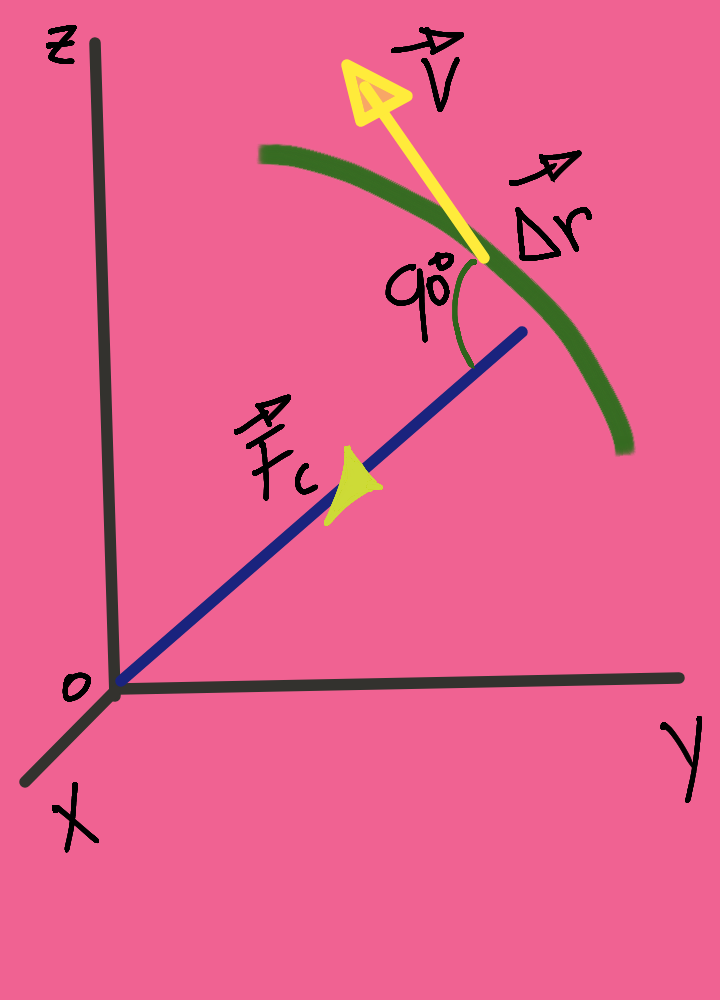# Kinetic energy

In this part of the physics he explains to us as a particle or mass the (x)th is at rest, which this one is under observation of a system of reference inertia, since it is demonstrated that the force is constant (F), which this one acquires an acceleration in form vectorial (a), in the same sense as part of his orientation, which at the same time stable the direction of the force applied in her.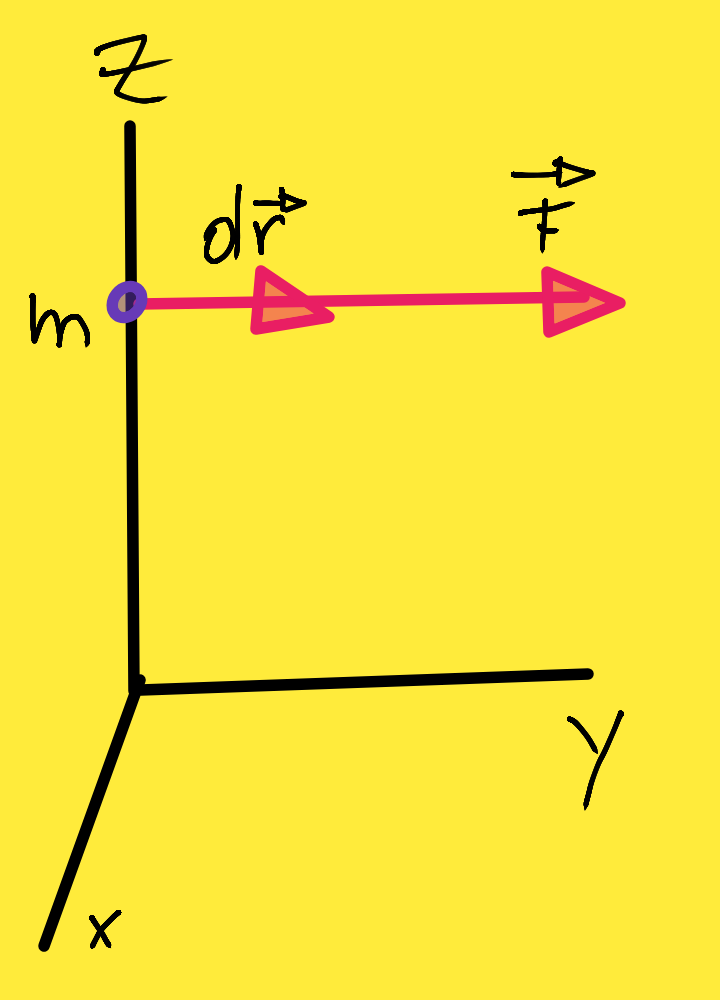Theorem of the alive forces in a system of particles.

Reading friend already trained in center of the content in this theorem shows us like in a particle or mass (m), on this one it operates a species of very singular force, since it can have certain speed in each of the masses to study, in what it represents that for each of the mass it operates a force, as part of location of the system of force, in which the interaction is reflected inform the particle doing very fascinatingly this phenomena.

Theorem of forces live: we will call kinetic energy of a system of material points to the sum of the kinetic energies of the different material points, to the sums of the energies kinetics of the different points that constitute the system. Each of these points is submitted to exterior and interior forces to the system and the work of all of them in a displacement of the point is equal to the increase of his kinetic energy. Information consulted in Initiation to the physics - Page 119 for Julián Fernández Ferrer, ‎Marcos Pujal Carrera, 1992.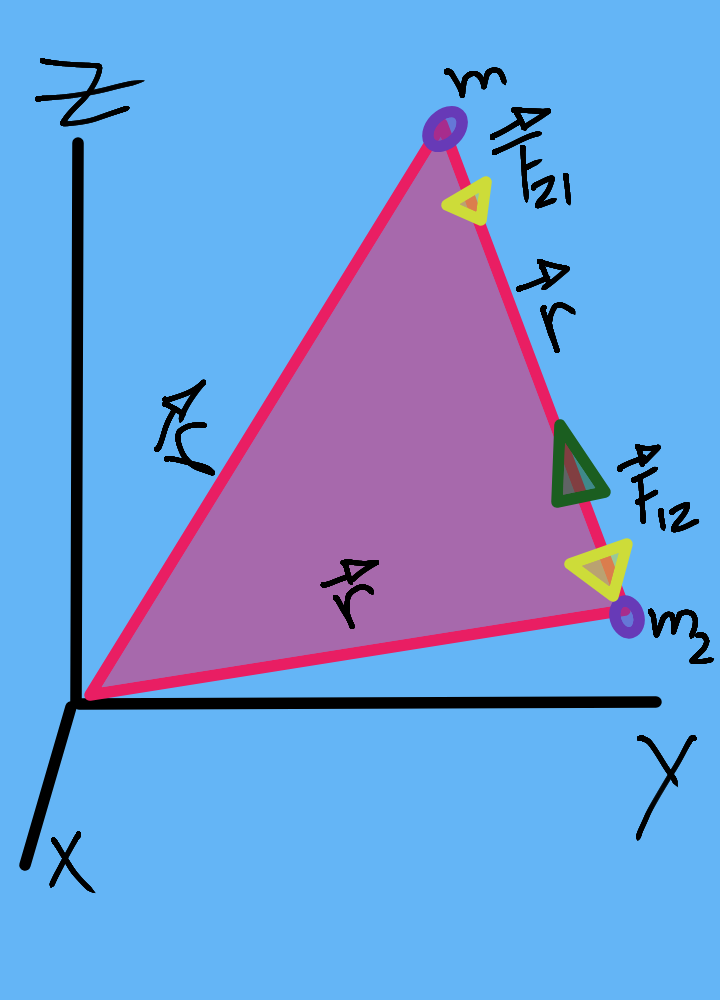The work realized by the clear force, this would be the sum of all the forces applied for one of the particle, this one goes to be equal to the change that experiences the kinetic energy of the above mentioned particle, where we have the following mathematical model:

W = ΔEc = Ec2 – Ec1

The most singular thing the case is under the scheme the mechanics newtoniana we have the following resultant one using the mathematical model of integral, the theorem of the alive forces for a particle: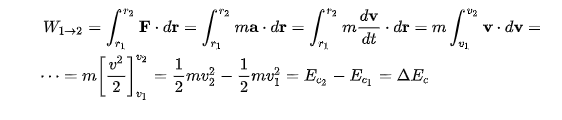Which shows us that Ec Ξ ½ mv2, which represents the cinematic energy of the individual or mass for r1 and r2, of equal way applying the use of vectors of position of each of them up to contemplating his final trip, for the action of the force exercised by them.

Slightly very important that also we give to have knowledge, it is that the energy measures itself in the same units as the work, like that the international unit of energy is the July, in such a way that it is a part of the kinetic energy, since this is the form of energy associated with the movement and his value which shows us with more felt the relation of force and movement: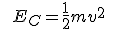Initiation to the physics - Page 119 for Julián Fernández Ferrer, ‎Marcos Pujal Carrera, 1992.

 General physics - Page 155 for Santiago Burbano, 2003.